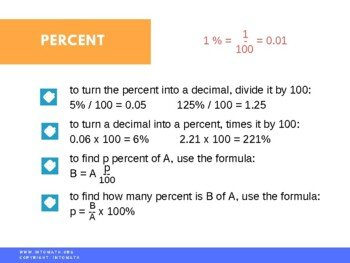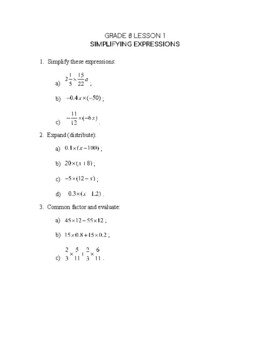CA\$2.00

In this lesson we are discussing two topics: square root of a number and percent.

A square root of a number is a number that has been squared to get the number under the square root.

For example, the √4 is 2, because 2 x 2 = 4.

Watch the video lesson to see the examples and learn why we cannot take a square root of a negative number.

We have already discussed the concept of percent in our previous lessons. However, since it is a very commonly used concept, we will go over it again in more detail.